SSAT Upper Level Math : Parallel Lines

Example Questions

Example Question #21 : Parallel Lines

Which of the following lines is parallel to the line with the equation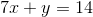?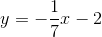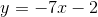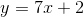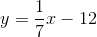Explanation:

Parallel lines have the same slope. First, put the given equation inform to find its slope.Subtract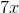from both sides of the equation: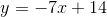The given line has a slope of, so the correct answer will also have a slope of. This means that the correct answer choice is.

Example Question #22 : Parallel Lines

Which of the following is a line that is parallel to the line with the equation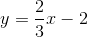?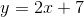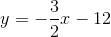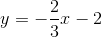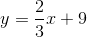Explanation:

For two lines to be parallel, their slopes must be the same. Since the slope of the given line is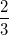, the line that is parallel to it must also have a slope of.

Example Question #23 : Parallel Lines

Linehas the equation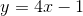. If lineis parallel to line, what is the slope of line?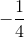Explanation:

Linemust have the same slope as lineto be parallel with it, so the slope of linemust be. You can tell that the slope of lineisbecause it is inform, and the value ofis the slope.

Example Question #14 : Coordinate Geometry

What is the slope of a line parallel to the line: -15x + 5y = 30 ?

30

3

1/3

-15

3

Explanation:

First, put the equation in slope-intercept form: y = 3x + 6. From there we can see the slope of this line is 3 and since the slope of any line parallel to another line is the same, the slope will also be 3.

Example Question #15 : Coordinate Geometry

What is the slope of any line parallel to –6x + 5y = 12?

6

6/5

5/6

12/5

12

6/5

Explanation:

This problem requires an understanding of the makeup of an equation of a line.  This problem gives an equation of a line in y = mx + b form, but we will need to algebraically manipulate the equation to determine its slope.  Once we have determined the slope of the line given we can determine the slope of any line parallel to it, becasue parallel lines have identical slopes.  By dividing both sides of the equation by 5, we are able to obtain an equation for this line that is in a more recognizable y = mx + b form. The equation of the line then becomes y = 6/5x + 12/5, we can see that the slope of this line is 6/5.

Example Question #16 : Coordinate Geometry

What is the slope of a line that is parallel to the line 11x + 4y - 2 = 9 – 4x  ?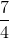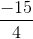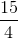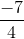Explanation:

We rearrange the line to express it in slope intercept form.

Any line parallel to this original line will have the same slope.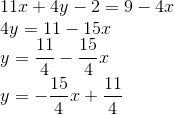Example Question #17 : Coordinate Geometry

In the standard (x, y) coordinate plane, what is the slope of a line parallel to the line with equation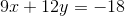?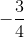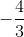Explanation:

Parallel lines will have equal slopes. To solve, we simply need to rearrange the given equation into slope-intercept form to find its slope.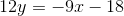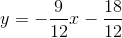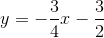The slope of the given line is. Any lines that run parallel to the given line will also have a slope of.

Example Question #18 : Coordinate Geometry

What is the slope of a line that is parallel to the line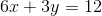?Explanation:

Parallel lines have the same slope. The question requires you to find the slope of the given function. The best way to do this is to put the equation in slope-intercept form (y = mx + b) by solving for y.

First subtract 6x on both sides to get 3y = –6x + 12.

Then divide each term by 3 to get y = –2x + 4.

In the form y = mx + b, m represents the slope. So the coefficient of the x term is the slope, and –2 is the correct answer.

Example Question #24 : Parallel Lines

Lineis defined by the equation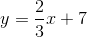. If Lineis parallel to Line, what is the slope of Line?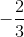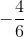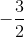Explanation:

Any line that is parallel to a linemust have the same slope. Since Linehas a slope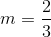, Linemust also have a slopeExample Question #25 : Parallel Lines

Which of the following is a line that is parallel to the line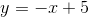?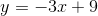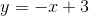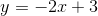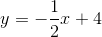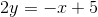For a given line, a parallel line must have the same slope. Given the answer choices above, onlyhas the same slope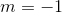.